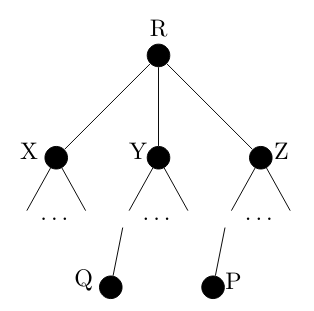# GATE | GATE CS 2021 | Set 2 | Question 55

• Last Updated : 23 May, 2021

Consider a computer network using the distance vector routing algorithm in its network layer. The partial topology of the network is shown below.The objective is to find the shortest-cost path from the router R to routers P and Q. Assume that R does not initially know the shortest routes to P and Q. Assume that R has three neighbouring routers denoted as X, Y and Z. During one iteration, R measures its distance to its neighbours X, Y, and Z as 3, 2 and 5, respectively. Router R gets routing vectors from its neighbours that indicate that the distance to router P from routers X, Y and Z are 7, 6 and 5, respectively. The routing vector also indicates that the distance to router Q from routers X, Y and Z are 4, 6 and 8 respectively. Which of the following statement(s) is/are correct with respect to the new routing table o R, after updation during this iteration?
(A) The distance from R to P will be stored as 10
(B) The distance from R to Q will be stored as 7
(C) The next hop router for a packet from R to P is Y
(D) The next hop router for a packet from R to Q is Z

Explanation: Given R gets the distance vector (3,2,5)

After the one iteration distance vector from X to P, Y to P, and Z to P is (7, 6, 5) respectively

The distance vector from R to P via X Y Z is (3+7, 2+6, 5+5) =(10, 8, 10)

So Take minimum distance from R to P which is 8 via Y

After the iteration distance vector from X to Q, Y to Q, Z to Q is ( 4, 6, 8) respectively

The distance vector from R to Q via X Y Z is (3+4, 2+6, 5+8) = (7, 8 13)

So Take minimum distance from R to Q which is 7 via X.

Quiz of this Question

My Personal Notes arrow_drop_up
Recommended Articles
Page :